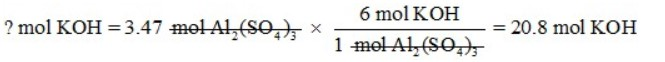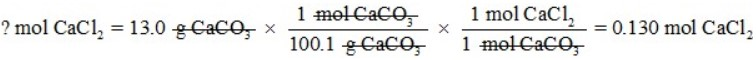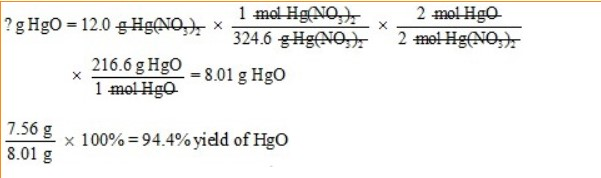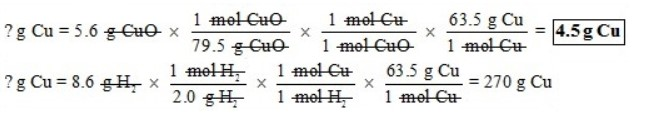# Stoichiometry Review

Stoichiometry is the study of quantity relationships in a chemical reaction. You are always given information about one substance in the reaction and asked for information about another substance. You “make the switch” between the two substances using the mole ratio. When a reaction is carried out in a laboratory, the amount of product is called the actual yield. The amount of product expected to be formed based on working the problem out mathematically is called the theoretical yield. To calculate the percent yield, you divide the actual yield by the theoretical yield, and then multiply by 100 percent. When looking at a chemical reaction, the reactant that runs out first is called the limiting reactant, and the reactant that has some left over is called the excess reactant.

Word Bank:

actual                                        excess                                          limiting
mole                                         theoretical                                   stoichiometry

Work each of the following problems showing all work. These problems are representative of each type of problem worked in this unit.

1. How many moles of potassium hydroxide are needed to completely react with 3.47 moles of aluminum sulfate according to the following BALANCED equation?

6 KOH + Al2(SO4)3 → 2 Al(OH)3 + 3 K2SO42. Calcium carbonate and sodium chloride react to produce sodium carbonate and calcium chloride according to the following BALANCED equation. How many moles of calcium chloride will be produced if 13.0 g of calcium carbonate are reacted?

CaCO3 + 2 NaCl → Na2CO3 + CaCl23. When mercury (II) nitrate is heated, it decomposes to form mercury (II) oxide, nitrogen dioxide, and oxygen gas according to the following BALANCED equation:

2 Hg(NO3)2 → 2 HgO + 4 NO2 + O2

a. How many grams of mercury (II) oxide will be produced if 27.0 g of mercury (II) nitrate react?b. How many moles of oxygen gas will be produced if 3.5 g of nitrogen dioxide are produced?c. When 12.0 g of mercury (II) nitrate are decomposed in the lab, it is found that 7.56 g of mercury (II) oxide are produced. What is the actual yield, the theoretical yield, and the percent yield?4. If 5.6 g of copper (II) oxide are reacted with 8.6 g of hydrogen according to the following BALANCED reaction, how many grams of copper metal will be produced?

CuO + H2 → Cu + H2OFill in the blanks. Highlight the sentence to reveal the answers.

The limiting reactant is CuO, and the excess reactant is H2O .

source